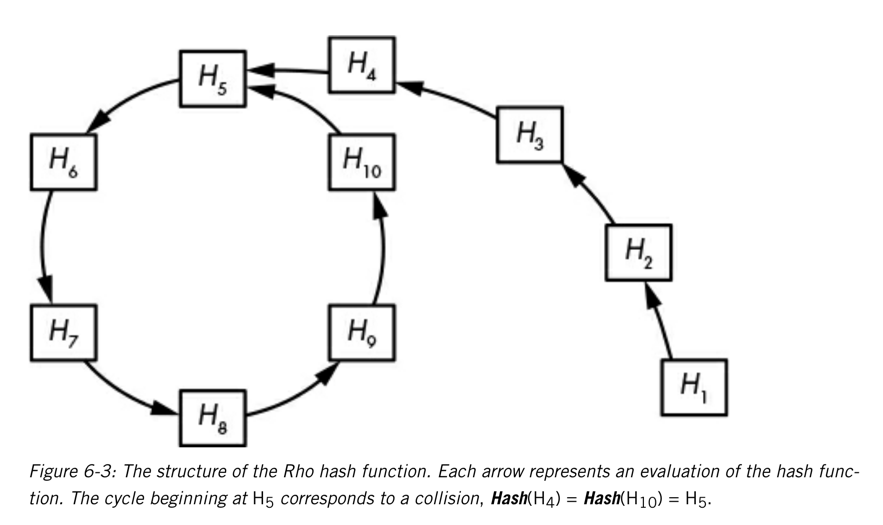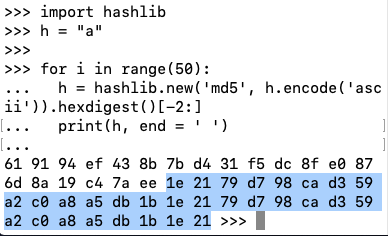C 106: The Rho Method (30 pts)

Background

The Rho method is used to find hash collisions without requiring a lot of RAM. The idea is to keep hashing the result of the previous hash operation, the same way you do when calculating password hashes with many rounds.

In this project, we won't conserve RAM, but just find cycles in hash functions.

If you hash a starting value, and then hash that hash, and repeat this process, you don't keep getting different numbers forever. Eventually you get a repeated value, and after that all further values are repeats, going in a loop, as shown below.Figure from Serious Cryptography

What You Need

Any computer with Python 3.

Calculating a MD5 Hash

In Python, execute this code, as shown below.
import hashlib
print(hashlib.new('md5', "a".encode('ascii')).hexdigest())
The md5 hash appears, starting with 0cc, as shown below.Checking with an online MD5 calculator shows that this hash is correct, as shown below.Truncating the Hash

To make a very simple case, we'll keep only the right two characters of the hash.

In Python, execute this code, as shown below.

import hashlib
print(hashlib.new('md5', "a".encode('ascii')).hexdigest()[-2:])
The result is 61, the last two characters in the hash, as shown below.Iterated Hashing

Now we'll repeat the hash function many times. There are 256 possible hash values, so you might expect the sequence to go for 100 or more values without repeating.

In Python, execute this code, as shown below.

import hashlib
h = "a"

for i in range(50):
h = hashlib.new('md5', h.encode('ascii')).hexdigest()[-2:]
print(h, end = ' ')
Adjusting the width of the window makes it easy to see when the values start repeating, as shown below.C 106.1: Different Starting Value (5 pts)

Start the truncated hash you used above with an initial string of "password" instead of "a".

The first repeated hash value is the flag.

C 106.2: Three Characters (10 pts)

Keep the last three characters of the MD5 hash. Use an initial string of "password".

The first repeated hash value is the flag.

C 106.3: 32-bit Hash (15 pts)

Keep the last eight characters of the MD5 hash (a total of 32 bits). Use an initial string of "password".

The first repeated hash value is the flag.

Posted 2-26-19
Ported to new scoring system 7-11-19